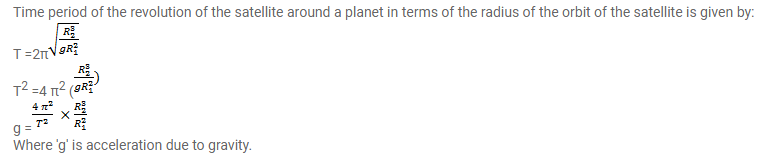# The radius of a planet is R,

Question:

The radius of a planet is $\mathrm{R}$, and a satellite revolves round it in a circle of radius $\mathrm{R} 2$. The time period of revolution is $\mathrm{T}$. Find the acceleration due to the gravitation of the planet at its surface.

Solution: